# Difference of Normals Based Segmentation

In this tutorial we will learn how to use Difference of Normals features, implemented in the pcl::DifferenceOfNormalsEstimation class, for scale-based segmentation of unorganized point clouds.

This algorithm performs a scale based segmentation of the given input point cloud, finding points that belong within the scale parameters given.

## Theoretical Primer

The Difference of Normals (DoN) provides a computationally efficient, multi-scale approach to processing large unorganized 3D point clouds. The idea is very simple in concept, and yet surprisingly effective in the segmentation of scenes with a wide variation of scale. For each point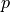in a pointcloud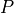, two unit point normals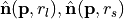are estimated with different radii,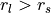. The normalized (vector) difference of these point normals defines the operator.

Formally the Difference of Normals operator is defined,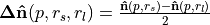where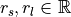,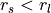, and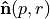is the surface normal estimate at point, given the support radius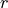. Notice, the response of the operator is a normalized vector field, and is thus orientable (the resulting direction is a key feature), however the operator’s norm often provides an easier quantity to work with, and is always in the range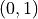.

The primary motivation behind DoN is the observation that surface normals estimated at any given radius reflect the underlying geometry of the surface at the scale of the support radius. Although there are many different methods of estimating the surface normals, normals are always estimated with a support radius (or via a fixed number of neighbours). This support radius determines the scale in the surface structure which the normal represents.

The above diagram illustrates this effect in 1D. Normals,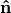, and tangents,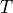, estimated with a small support radius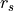are affected by small-scale surface structure (and similarly by noise). On the other hand, normals and tangent planes estimated with a large support radius $r_l$ are less affected by small-scale structure, and represent the geometry of larger scale surface structures. In fact a similar set of features is seen in the DoN feature vectors for real-world street curbs in a LiDAR image shown below.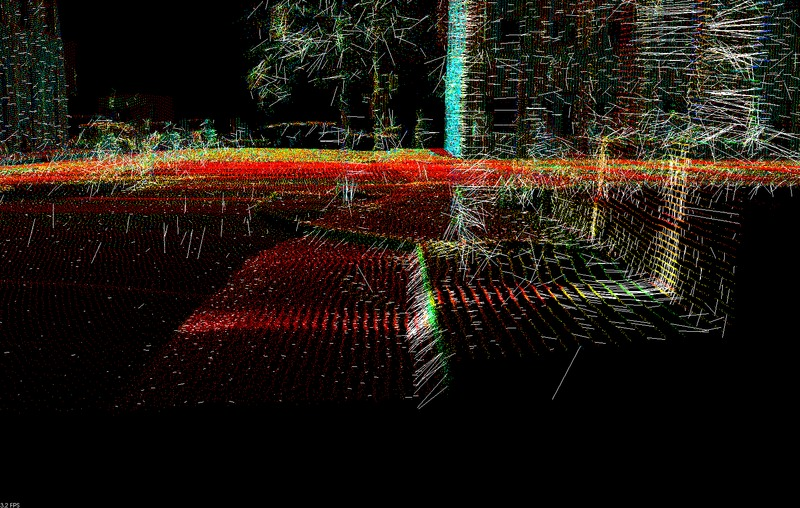Closeup of the DoN feature vectors calculated for a LiDAR pointcloud of a street curb.

For more comprehensive information, please refer to the article [DON2012].

## Using Difference of Normals for Segmentation

For segmentation we simply perform the following:

1. Estimate the normals for every point using a large support radius of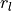2. Estimate the normals for every point using the small support radius of3. For every point the normalized difference of normals for every point, as defined above.

4. Filter the resulting vector field to isolate points belonging to the scale/region of interest.

## The Data Set

For this tutorial we suggest the use of publicly available (creative commons licensed) urban LiDAR data from the [KITTI] project. This data is collected from a Velodyne LiDAR scanner mounted on a car, for the purpose of evaluating self-driving cars. To convert the data set to PCL compatible point clouds please see [KITTIPCL]. Examples and an example data set will be posted here in future as part of the tutorial.

## The Code

Next what you need to do is to create a file don_segmentation.cpp in any editor you prefer and copy the following code inside of it:

  1/**
2 * @file don_segmentation.cpp
3 * Difference of Normals Example for PCL Segmentation Tutorials.
4 *
5 * @author Yani Ioannou
6 * @date 2012-09-24
7 */
8#include <string>
9
10#include <pcl/point_types.h>
11#include <pcl/io/pcd_io.h>
12#include <pcl/search/organized.h>
13#include <pcl/search/kdtree.h>
14#include <pcl/features/normal_3d_omp.h>
15#include <pcl/filters/conditional_removal.h>
16#include <pcl/segmentation/extract_clusters.h>
17
18#include <pcl/features/don.h>
19
20using namespace pcl;
21
22int
23main (int argc, char *argv[])
24{
25  ///The smallest scale to use in the DoN filter.
26  double scale1;
27
28  ///The largest scale to use in the DoN filter.
29  double scale2;
30
31  ///The minimum DoN magnitude to threshold by
32  double threshold;
33
34  ///segment scene into clusters with given distance tolerance using euclidean clustering
36
37  if (argc < 6)
38  {
39    std::cerr << "usage: " << argv << " inputfile smallscale largescale threshold segradius" << std::endl;
40    exit (EXIT_FAILURE);
41  }
42
43  /// the file to read from.
44  std::string infile = argv;
45  /// small scale
46  std::istringstream (argv) >> scale1;
47  /// large scale
48  std::istringstream (argv) >> scale2;
49  std::istringstream (argv) >> threshold;   // threshold for DoN magnitude
51
52  // Load cloud in blob format
53  pcl::PCLPointCloud2 blob;
55  pcl::PointCloud<PointXYZRGB>::Ptr cloud (new pcl::PointCloud<PointXYZRGB>);
56  pcl::fromPCLPointCloud2 (blob, *cloud);
57
58  // Create a search tree, use KDTreee for non-organized data.
59  pcl::search::Search<PointXYZRGB>::Ptr tree;
60  if (cloud->isOrganized ())
61  {
62    tree.reset (new pcl::search::OrganizedNeighbor<PointXYZRGB> ());
63  }
64  else
65  {
66    tree.reset (new pcl::search::KdTree<PointXYZRGB> (false));
67  }
68
69  // Set the input pointcloud for the search tree
70  tree->setInputCloud (cloud);
71
72  if (scale1 >= scale2)
73  {
74    std::cerr << "Error: Large scale must be > small scale!" << std::endl;
75    exit (EXIT_FAILURE);
76  }
77
78  // Compute normals using both small and large scales at each point
79  pcl::NormalEstimationOMP<PointXYZRGB, PointNormal> ne;
80  ne.setInputCloud (cloud);
81  ne.setSearchMethod (tree);
82
83  /**
84   * NOTE: setting viewpoint is very important, so that we can ensure
85   * normals are all pointed in the same direction!
86   */
87  ne.setViewPoint (std::numeric_limits<float>::max (), std::numeric_limits<float>::max (), std::numeric_limits<float>::max ());
88
89  // calculate normals with the small scale
90  std::cout << "Calculating normals for scale..." << scale1 << std::endl;
91  pcl::PointCloud<PointNormal>::Ptr normals_small_scale (new pcl::PointCloud<PointNormal>);
92
94  ne.compute (*normals_small_scale);
95
96  // calculate normals with the large scale
97  std::cout << "Calculating normals for scale..." << scale2 << std::endl;
98  pcl::PointCloud<PointNormal>::Ptr normals_large_scale (new pcl::PointCloud<PointNormal>);
99
101  ne.compute (*normals_large_scale);
102
103  // Create output cloud for DoN results
104  PointCloud<PointNormal>::Ptr doncloud (new pcl::PointCloud<PointNormal>);
105  copyPointCloud (*cloud, *doncloud);
106
107  std::cout << "Calculating DoN... " << std::endl;
108  // Create DoN operator
109  pcl::DifferenceOfNormalsEstimation<PointXYZRGB, PointNormal, PointNormal> don;
110  don.setInputCloud (cloud);
111  don.setNormalScaleLarge (normals_large_scale);
112  don.setNormalScaleSmall (normals_small_scale);
113
114  if (!don.initCompute ())
115  {
116    std::cerr << "Error: Could not initialize DoN feature operator" << std::endl;
117    exit (EXIT_FAILURE);
118  }
119
120  // Compute DoN
121  don.computeFeature (*doncloud);
122
123  // Save DoN features
124  pcl::PCDWriter writer;
125  writer.write<pcl::PointNormal> ("don.pcd", *doncloud, false);
126
127  // Filter by magnitude
128  std::cout << "Filtering out DoN mag <= " << threshold << "..." << std::endl;
129
130  // Build the condition for filtering
131  pcl::ConditionOr<PointNormal>::Ptr range_cond (
132    new pcl::ConditionOr<PointNormal> ()
133    );
135                               new pcl::FieldComparison<PointNormal> ("curvature", pcl::ComparisonOps::GT, threshold))
136                             );
137  // Build the filter
138  pcl::ConditionalRemoval<PointNormal> condrem;
139  condrem.setCondition (range_cond);
140  condrem.setInputCloud (doncloud);
141
142  pcl::PointCloud<PointNormal>::Ptr doncloud_filtered (new pcl::PointCloud<PointNormal>);
143
144  // Apply filter
145  condrem.filter (*doncloud_filtered);
146
147  doncloud = doncloud_filtered;
148
149  // Save filtered output
150  std::cout << "Filtered Pointcloud: " << doncloud->size () << " data points." << std::endl;
151
152  writer.write<pcl::PointNormal> ("don_filtered.pcd", *doncloud, false);
153
154  // Filter by magnitude
155  std::cout << "Clustering using EuclideanClusterExtraction with tolerance <= " << segradius << "..." << std::endl;
156
157  pcl::search::KdTree<PointNormal>::Ptr segtree (new pcl::search::KdTree<PointNormal>);
158  segtree->setInputCloud (doncloud);
159
160  std::vector<pcl::PointIndices> cluster_indices;
161  pcl::EuclideanClusterExtraction<PointNormal> ec;
162
164  ec.setMinClusterSize (50);
165  ec.setMaxClusterSize (100000);
166  ec.setSearchMethod (segtree);
167  ec.setInputCloud (doncloud);
168  ec.extract (cluster_indices);
169
170  int j = 0;
171  for (const auto& cluster : cluster_indices)
172  {
173    pcl::PointCloud<PointNormal>::Ptr cloud_cluster_don (new pcl::PointCloud<PointNormal>);
174    for (const auto& idx : cluster.indices)
175    {
176      cloud_cluster_don->points.push_back ((*doncloud)[idx]);
177    }
178
179    cloud_cluster_don->width = cloud_cluster_don->size ();
180    cloud_cluster_don->height = 1;
181    cloud_cluster_don->is_dense = true;
182
183    //Save cluster
184    std::cout << "PointCloud representing the Cluster: " << cloud_cluster_don->size () << " data points." << std::endl;
185    std::stringstream ss;
186    ss << "don_cluster_" << j << ".pcd";
187    writer.write<pcl::PointNormal> (ss.str (), *cloud_cluster_don, false);
188    ++j;
189  }
190}


### Compiling and running the program

 1cmake_minimum_required(VERSION 3.5 FATAL_ERROR)
2
3project(don_segmentation)
4
5find_package(PCL 1.7 REQUIRED)
6
7include_directories(${PCL_INCLUDE_DIRS}) 8link_directories(${PCL_LIBRARY_DIRS})
9add_definitions(${PCL_DEFINITIONS}) 10 11add_executable (don_segmentation don_segmentation.cpp) 12target_link_libraries (don_segmentation${PCL_LIBRARIES})


Create a build directory, and build the executable:

$mkdir build$ cd build
$cmake ..$ make


After you have made the executable, you can run it. Simply run:

\$ ./don_segmentation <inputfile> <smallscale> <largescale> <threshold> <segradius>


## The Explanation

  pcl::search::Search<PointXYZRGB>::Ptr tree;
if (cloud->isOrganized ())
{
tree.reset (new pcl::search::OrganizedNeighbor<PointXYZRGB> ());
}
else
{
tree.reset (new pcl::search::KdTree<PointXYZRGB> (false));
}

// Set the input pointcloud for the search tree
tree->setInputCloud (cloud);


We will skip the code for loading files and parsing command line arguments, and go straight to the first major PCL calls. For our later calls to calculate normals, we need to create a search tree. For organized data (i.e. a depth image), a much faster search tree is the OrganizedNeighbor search tree. For unorganized data, i.e. LiDAR scans, a KDTree is a good option.

  pcl::NormalEstimationOMP<PointXYZRGB, PointNormal> ne;
ne.setInputCloud (cloud);
ne.setSearchMethod (tree);

/**
* NOTE: setting viewpoint is very important, so that we can ensure
* normals are all pointed in the same direction!
*/
ne.setViewPoint (std::numeric_limits<float>::max (), std::numeric_limits<float>::max (), std::numeric_limits<float>::max ());

// calculate normals with the small scale


This is perhaps the most important section of code, estimating the normals. This is also the bottleneck computationally, and so we will use the pcl::NormalEstimationOMP class which makes use of OpenMP to use many threads to calculate the normal using the multiple cores found in most modern processors. We could also use the standard single-threaded class pcl::NormalEstimation, or even the GPU accelerated class pcl::gpu::NormalEstimation. Whatever class we use, it is important to set an arbitrary viewpoint to be used across all the normal calculations - this ensures that normals estimated at different scales share a consistent orientation.

Note

For information and examples on estimating normals, normal ambiguity, and the different normal estimation methods in PCL, please read the Estimating Surface Normals in a PointCloud tutorial.

  std::cout << "Calculating normals for scale..." << scale1 << std::endl;
pcl::PointCloud<PointNormal>::Ptr normals_small_scale (new pcl::PointCloud<PointNormal>);

ne.compute (*normals_small_scale);

// calculate normals with the large scale
std::cout << "Calculating normals for scale..." << scale2 << std::endl;
pcl::PointCloud<PointNormal>::Ptr normals_large_scale (new pcl::PointCloud<PointNormal>);

ne.compute (*normals_large_scale);


Next we calculate the normals using our normal estimation class for both the large and small radius. It is important to use the NormalEstimation.setRadiusSearch() method v.s. the NormalEstimation.setMaximumNeighbours() method or equivalent. If the normal estimate is restricted to a set number of neighbours, it may not be based on the complete surface of the given radius, and thus is not suitable for the Difference of Normals features.

Note

For large supporting radii in dense point clouds, calculating the normal would be a very computationally intensive task potentially utilizing thousands of points in the calculation, when hundreds are more than enough for an accurate estimate. A simple method to speed up the calculation is to uniformly subsample the pointcloud when doing a large radius search, see the full example code in the PCL distribution at examples/features/example_difference_of_normals.cpp for more details.

### Difference of Normals Feature Calculation

  PointCloud<PointNormal>::Ptr doncloud (new pcl::PointCloud<PointNormal>);
copyPointCloud (*cloud, *doncloud);


We can now perform the actual Difference of Normals feature calculation using our normal estimates. The Difference of Normals result is a vector field, so we initialize the point cloud to store the results in as a pcl::PointNormal point cloud, and copy the points from our input pointcloud over to it, so we have what may be regarded as an uninitialized vector field for our point cloud.

  pcl::DifferenceOfNormalsEstimation<PointXYZRGB, PointNormal, PointNormal> don;
don.setInputCloud (cloud);
don.setNormalScaleLarge (normals_large_scale);
don.setNormalScaleSmall (normals_small_scale);

if (!don.initCompute ())
{
std::cerr << "Error: Could not initialize DoN feature operator" << std::endl;
exit (EXIT_FAILURE);
}

// Compute DoN
don.computeFeature (*doncloud);


We instantiate a new pcl::DifferenceOfNormalsEstimation class to take care of calculating the Difference of Normals vector field.

The pcl::DifferenceOfNormalsEstimation class has 3 template parameters, the first corresponds to the input point cloud type, in this case pcl::PointXYZRGB, the second corresponds to the type of the normals estimated for the point cloud, in this case pcl::PointNormal, and the third corresponds to the vector field output type, in this case also pcl::PointNormal. Next we set the input point cloud and give both of the normals estimated for the point cloud, and check that the requirements for computing the features are satisfied using the pcl::DifferenceOfNormalsEstimation::initCompute() method. Finally we compute the features by calling the pcl::DifferenceOfNormalsEstimation::computeFeature() method.

Note

The pcl::DifferenceOfNormalsEstimation class expects the given point cloud and normal point clouds indices to match, i.e. the first point in the input point cloud’s normals should also be the first point in the two normal point clouds.

### Difference of Normals Based Filtering

While we now have a Difference of Normals vector field, we still have the complete point set. To begin the segmentation process, we must actually discriminate points based on their Difference of Normals vector result. There are a number of common quantities you may want to try filtering by:

Quantity

PointNormal Field

Description

Usage Scenario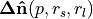float normal

DoN vector

Filtering points by relative DoN angle.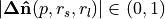float curvature

DoN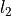norm

Filtering points by scale membership, large magnitude indicates point has a strong response at then given scale parameters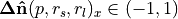,

float normal

DoN vector x component

Filtering points by orientable scale, i.e. building facades with large large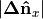and/or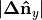and small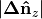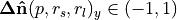,

float normal

DoN vector y component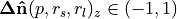,

float normal

DoN vector z component

In this example we will do a simple magnitude threshold, looking for objects of a scale regardless of their orientation in the scene. To do so, we must create a conditional filter:

  pcl::ConditionOr<PointNormal>::Ptr range_cond (
new pcl::ConditionOr<PointNormal> ()
);
new pcl::FieldComparison<PointNormal> ("curvature", pcl::ComparisonOps::GT, threshold))
);
// Build the filter
pcl::ConditionalRemoval<PointNormal> condrem;
condrem.setCondition (range_cond);
condrem.setInputCloud (doncloud);

pcl::PointCloud<PointNormal>::Ptr doncloud_filtered (new pcl::PointCloud<PointNormal>);

// Apply filter
condrem.filter (*doncloud_filtered);


After we apply the filter we are left with a reduced pointcloud consisting of the points with a strong response with the given scale parameters.

Note

### Clustering the Results

  std::cout << "Clustering using EuclideanClusterExtraction with tolerance <= " << segradius << "..." << std::endl;

pcl::search::KdTree<PointNormal>::Ptr segtree (new pcl::search::KdTree<PointNormal>);
segtree->setInputCloud (doncloud);

std::vector<pcl::PointIndices> cluster_indices;
pcl::EuclideanClusterExtraction<PointNormal> ec;

ec.setMinClusterSize (50);
ec.setMaxClusterSize (100000);
ec.setSearchMethod (segtree);
ec.setInputCloud (doncloud);
ec.extract (cluster_indices);


Finally, we are usually left with a number of objects or regions with good isolation, allowing us to use a simple clustering algorithm to segment the results. In this example we used Euclidean Clustering with a threshold equal to the small radius parameter.

Note

After the segmentation the cloud viewer window will be opened and you will see something similar to those images: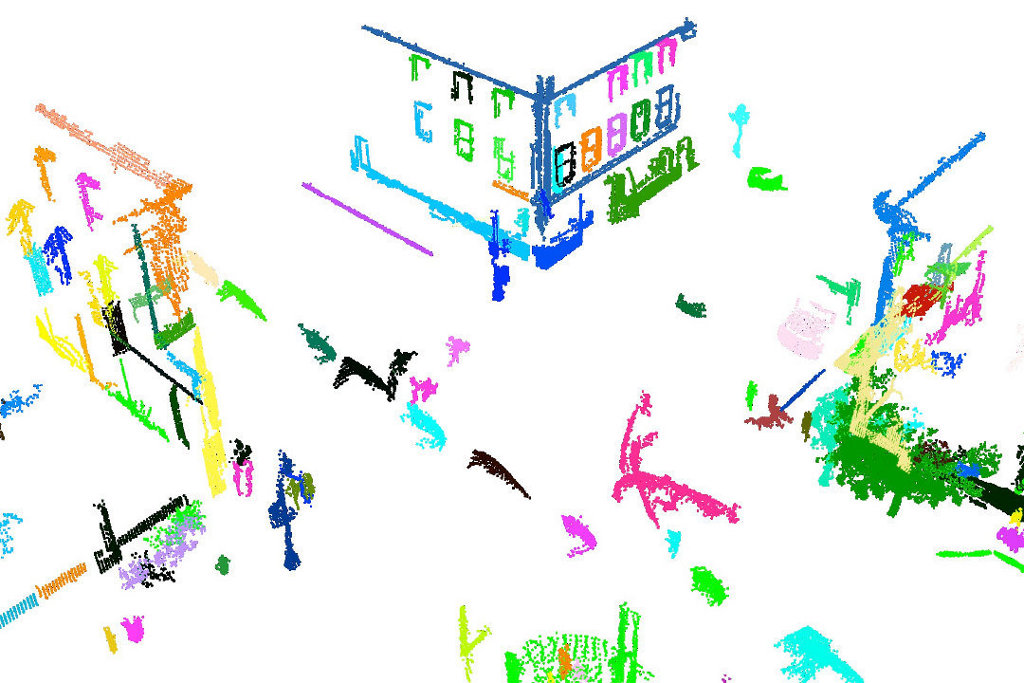### References/Further Information

[DON2012]

“Difference of Normals as a Multi-Scale Operator in Unorganized Point Clouds” <http://arxiv.org/abs/1209.1759>.

Note

@ARTICLE{2012arXiv1209.1759I, author = {{Ioannou}, Y. and {Taati}, B. and {Harrap}, R. and {Greenspan}, M.}, title = “{Difference of Normals as a Multi-Scale Operator in Unorganized Point Clouds}”, journal = {ArXiv e-prints}, archivePrefix = “arXiv”, eprint = {1209.1759}, primaryClass = “cs.CV”, keywords = {Computer Science - Computer Vision and Pattern Recognition}, year = 2012, month = sep, }

[KITTI]

“The KITTI Vision Benchmark Suite” <http://www.cvlibs.net/datasets/kitti/>.

[KITTIPCL]

“KITTI PCL Toolkit” <https://github.com/yanii/kitti-pcl>